# Vectors Worksheet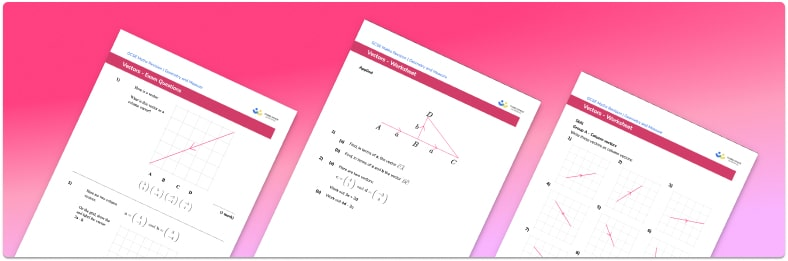• Section 1 of the vector worksheet contains 60 skills-based vector questions, in 3 groups to support differentiation
• Section 2 contains 4 applied vector questions with a mix of worded problems and deeper problem solving questions
• Section 3 contains 4 foundation and higher level GCSE exam questions on vectors
• Answers and a mark scheme for all questions are provided
• Questions follow variation theory with plenty of opportunities for students to work independently at their own level
• All questions created by fully qualified expert secondary maths teachers
• Suitable for GCSE maths revision for AQA, OCR and Edexcel exam boards

• This field is for validation purposes and should be left unchanged.

You can unsubscribe at any time (each email we send will contain an easy way to unsubscribe). To find out more about how we use your data, see our privacy policy.

### Vectors at a glance

A vector describes a movement from one point to another and has both a magnitude and a direction.

We can use vector addition and subtraction to create a pathway from one point to another. Adding and subtracting vectors involves adding or subtracting the different components of a vector. We can also multiply a vector by a scalar to make it shorter or longer. If a scalar is negative it will reverse the direction of the vector but otherwise scalar multiples have no effect on the direction of a vector.

We can calculate the magnitude of a vector using Pythagoras’ theorem and a unit vector has magnitude 1.

Vector problems often involve midpoints of lines, fractions of lines and ratios between different parts of lines. We have to think carefully about how the information we are presented with applies to the problem and the clearest way to do this is usually to create a labelled diagram.

Looking forward, students can then progress to additional geometry worksheets, for example, an area of compound shapes worksheet or how to work out perimeter worksheet.For more teaching and learning support on Geometry our GCSE maths lessons provide step by step support for all GCSE maths concepts.

## Related worksheets

Simple Vectors Worksheet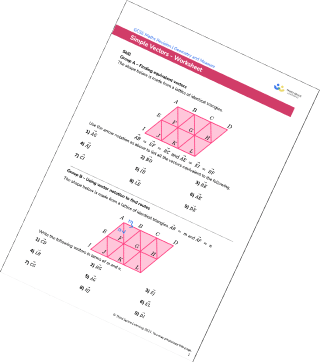Vector Problems Worksheet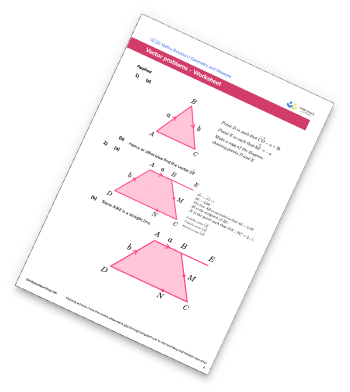Congruent Triangles Worksheet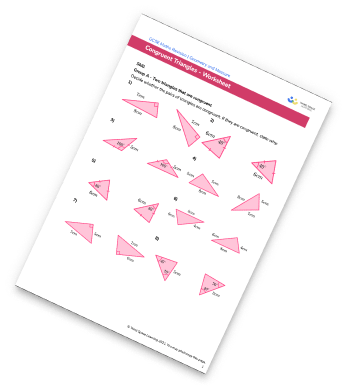Volume Worksheet – How To Calculate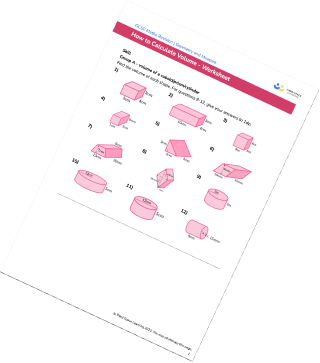## Do you have KS4 students who need more focused attention to succeed at GCSE?There will be students in your class who require individual attention to help them succeed in their maths GCSEs. In a class of 30, it’s not always easy to provide.

Help your students feel confident with exam-style questions and the strategies they’ll need to answer them correctly with our dedicated GCSE maths revision programme.

Lessons are selected to provide support where each student needs it most, and specially-trained GCSE maths tutors adapt the pitch and pace of each lesson. This ensures a personalised revision programme that raises grades and boosts confidence.

Find out more